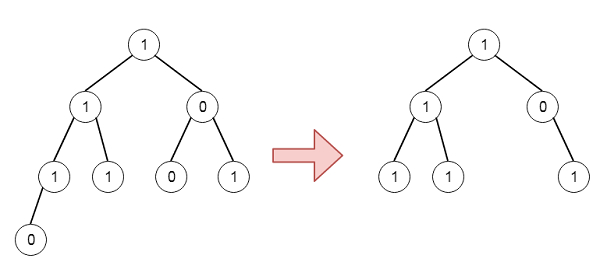# Binary Tree Pruning in C++

Suppose we have the head node root of a binary tree, where additionally every node's value is either a 0 or a 1. We have to find the same tree where every subtree not containing a 1 has been deleted. So if the tree is like −To solve this, we will follow these steps −

• Define a recursive method solve(), this will take the node. the method will be like −

• If node is null, then return null

• left of node := solve(left of node)

• right of node := solve(right of node)

• if left of node is null and right of node is also null and node value is 0, then return null

• return node

Let us see the following implementation to get better understanding −

## Example

Live Demo

#include <bits/stdc++.h>
using namespace std;
class TreeNode{
public:
int val;
TreeNode *left, *right;
TreeNode(int data){
val = data;
left = NULL;
right = NULL;
}
};
void insert(TreeNode **root, int val){
queue<TreeNode*> q;
q.push(*root);
while(q.size()){
TreeNode *temp = q.front();
q.pop();
if(!temp->left){
temp->left = new TreeNode(val);
return;
}else{
q.push(temp->left);
}
if(!temp->right){
temp->right = new TreeNode(val);
return;
}else{
q.push(temp->right);
}
}
}
TreeNode *make_tree(vector<int> v){
TreeNode *root = new TreeNode(v);
for(int i = 1; i<v.size(); i++){
insert(&root, v[i]);
}
return root;
}
void tree_level_trav(TreeNode*root){
if (root == NULL) return;
cout << "[";
queue<TreeNode *> q;
TreeNode *curr;
q.push(root);
q.push(NULL);
while (q.size() > 1) {
curr = q.front();
q.pop();
if (curr == NULL){
q.push(NULL);
} else {
if(curr->left)
q.push(curr->left);
if(curr->right)
q.push(curr->right);
if(curr == NULL){
cout << "null" << ", ";
}else{
cout << curr->val << ", ";
}
}
}
cout << "]"<<endl;
}
class Solution {
public:
TreeNode* pruneTree(TreeNode* node) {
if(!node)return NULL;
node->left = pruneTree(node->left);
node->right = pruneTree(node->right);
if(!node->left && !node->right && !node->val){
return NULL;
}
return node;
}
};
main(){
vector<int> v = {1,1,0,1,1,0,1,0};
TreeNode *root = make_tree(v);
Solution ob;
tree_level_trav(ob.pruneTree(root));
}

## Input

[1,1,0,1,1,0,1,0]

## Output

[1, 1, 0, 1, 1, 1, ]

Updated on: 02-May-2020

332 Views